# HOW TO MAKE 8 HALF-SQUARE TRIANGLES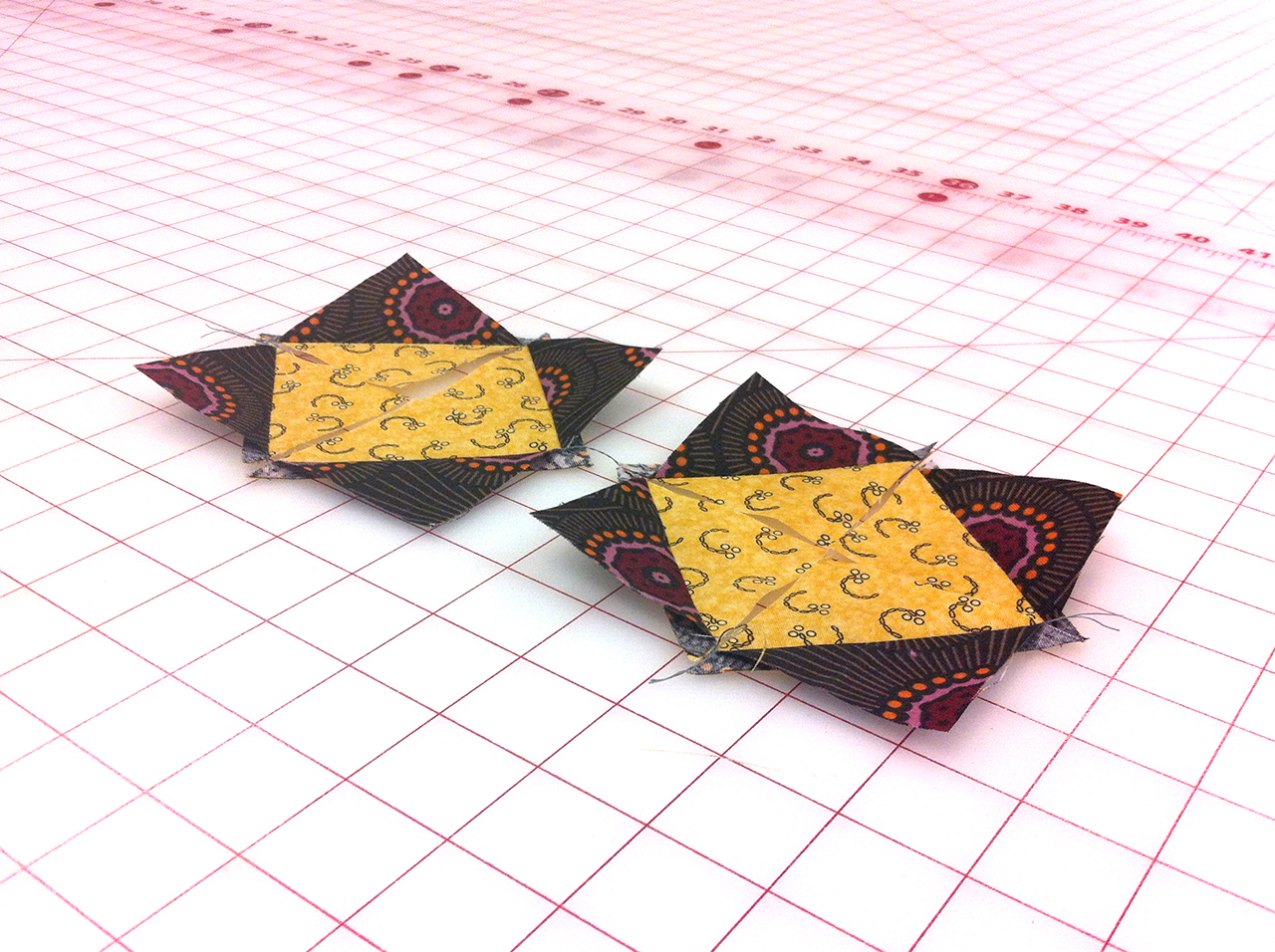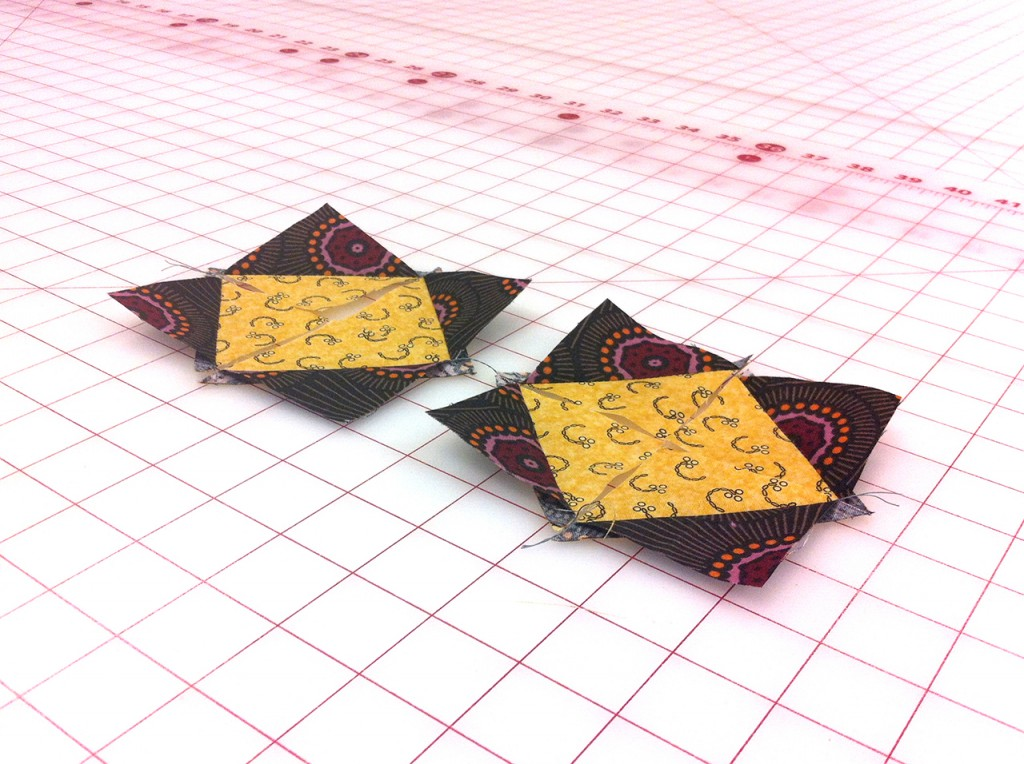In this tutorial we’ll show you an easy way to make 8 half square triangles at once. The cool thing about this method in comparison to the 4 at a time method is that the bias is where it’s supposed to be. Plus, your final edges are on the straight of grain.

Good ole math! Start with 5” squares and the half-square triangles are a bit over 2” and will end up being 1 1/2” once in the quilt. About 1” will be loss using this method.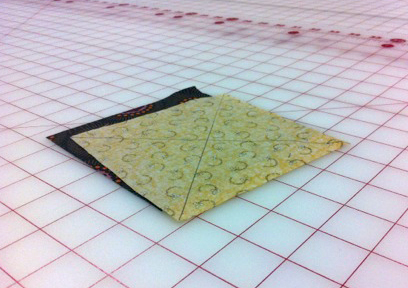Simply place two squares right side together and draw two lines diagonally on one of them like in the example above. Next sew 1/4” on both sides of each line, that’s four seams total.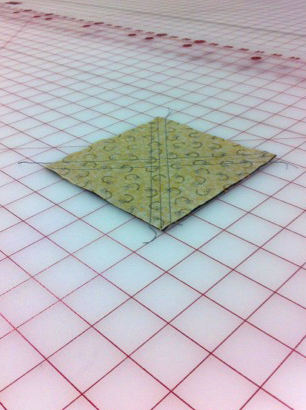Now, use the center line as a guide to cut a horizontal and vertical line.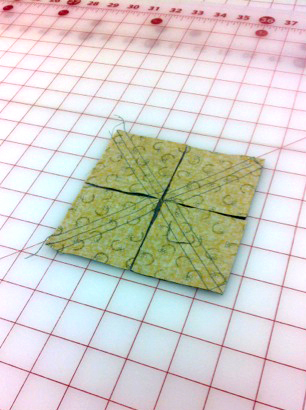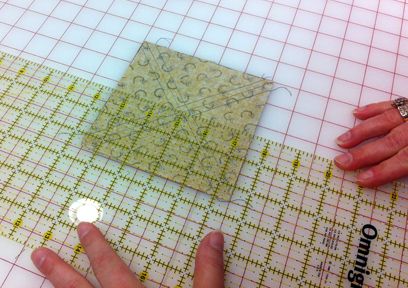Cut along the pencil lines and there you have it, a half-square triangle.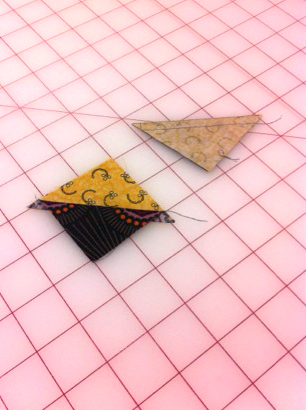All there is left to do is trim to size if needed.Stay tuned for more great tutorials, tips and tricks from AllBrands.com!